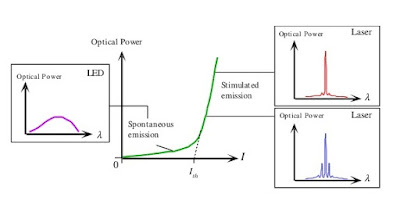## Page

### Power current characteristics of laser diode

To shown in the figure the output optic power versus forwarding input current characteristics is plotted in the figure for a typical laser diode.

To shown in the figure below the threshold current only spontaneous emission is emitted hence there is a small increase in optic power with drive current.

At threshold when lasing conditions are satisfied. At that time when the optical power increases sharply after the lasing threshold because of stimulated emission.

The lasing threshold optical gain is related by threshold current density for stimulated emission by the expression  is given below :

Gth =  β  Jth

Where β is constant for device structure.Power current characteristics of a laser diode
To shown in the figure the output optic power versus forwarding input current characteristics is plotted in the figure for a typical laser diode.

To shown in the figure below the threshold current only spontaneous emission is emitted hence there is a small increase in optic power with drive current.

At threshold when lasing conditions are satisfied. At that time when the optical power increases sharply after the lasing threshold because of stimulated emission.

The lasing threshold optical gain is related by threshold current density for stimulated emission by the expression  is given below :

Gth =  β  Jth

Where β is constant for device structure.Power current characteristics of a laser diode